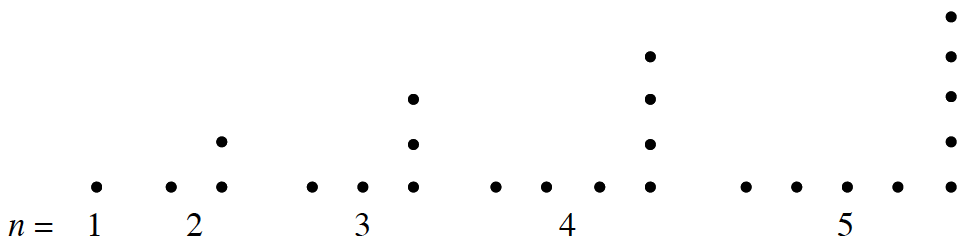### Home > CCA2 > Chapter Ch3 > Lesson 3.2.1 > Problem3-63

3-63.

Given that $n$ is the length of the bottom edge of the backward L-shaped figures below, what sequence is generated by the total number of dots in each figure? What is the $46^{\text{th}}$ term, or, $t(46)$ of this sequence? The $n^{\text{th}}$ term? Homework Help ✎Find a rule for the sequence. How does the figure number relate to parts in the shape?

 $n$ $\ \ \ 1\ \ \ \ 2\ \ \ \ ...$ $t\left(n\right)$ $\ \ \ 1\ \ \ \ 3\ \ \ \ ...$

Look at the two legs. One way to see figure 3, for example, is a row of 3 and a column of 2 above it (note: you can't count the dot in the corner twice). How would you analyze figure 4 in the same way?

Now use the idea to analyze the $n^{\text{th}}$ figure. If figure $3\ \text{is}\ 3+(3−1)$, and figure $4\ \text{is}\ 4+(4−1)$, what is the number of dots in figure $n$?

The $n^{\text{th}}\ \text{term}=n+(n−1)=2n−1$.

The $46^{\text{th}}\ \text{term}=46+(46−1)=46+45=91\ \text{dots}$.